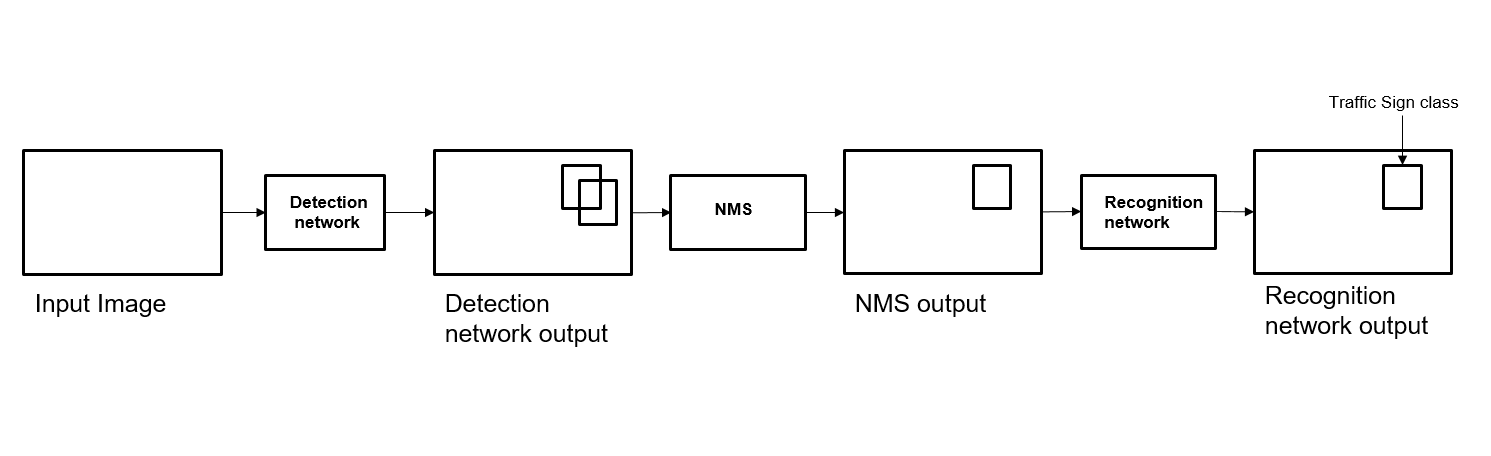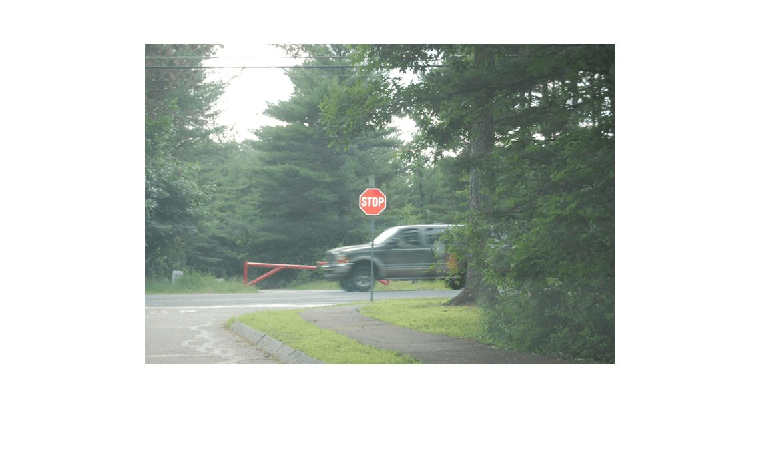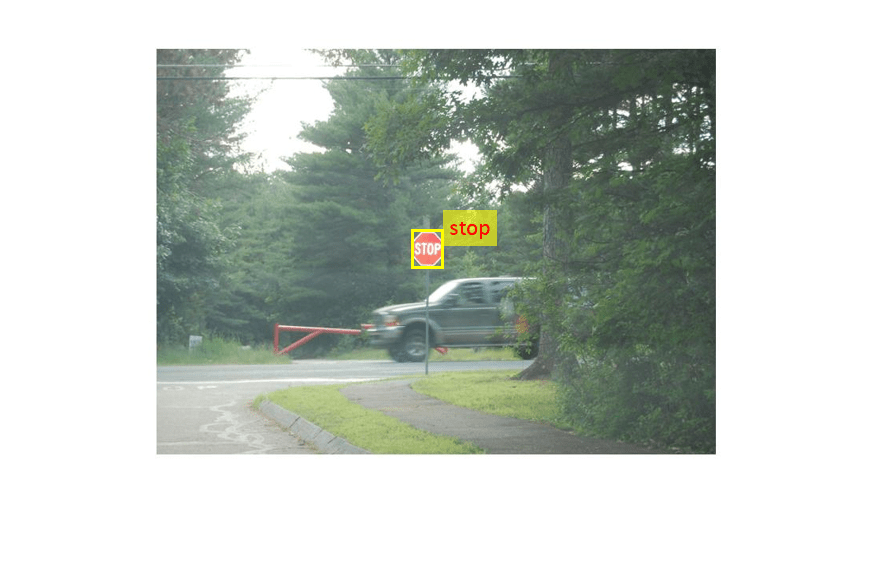# Traffic Sign Detection and Recognition

This example shows how to generate CUDA® MEX code for a traffic sign detection and recognition application that uses deep learning. Traffic sign detection and recognition is an important application for driver assistance systems, aiding and providing information to the driver about road signs.In this traffic sign detection and recognition example you perform three steps - detection, Non-Maximal Suppression (NMS), and recognition. First, the example detects the traffic signs on an input image by using an object detection network that is a variant of the You Only Look Once (YOLO) network. Then, overlapping detections are suppressed by using the NMS algorithm. Finally, the recognition network classifies the detected traffic signs.

### Third-Party Prerequisites

Required

This example generates CUDA MEX and has the following third-party requirements.

• CUDA® enabled NVIDIA® GPU and compatible driver.

Optional

For non-MEX builds such as static, dynamic libraries or executables, this example has the following additional requirements.

### Verify GPU Environment

Use the `coder.checkGpuInstall` function to verify that the compilers and libraries necessary for running this example are set up correctly.

```envCfg = coder.gpuEnvConfig('host'); envCfg.DeepLibTarget = 'cudnn'; envCfg.DeepCodegen = 1; envCfg.Quiet = 1; coder.checkGpuInstall(envCfg);```

### Detection and Recognition Networks

The detection network is trained in the Darknet framework and imported into MATLAB® for inference. Because the size of the traffic sign is relatively small with respect to that of the image and the number of training samples per class are fewer in the training data, all the traffic signs are considered as a single class for training the detection network.

The detection network divides the input image into a 7-by-7 grid. Each grid cell detects a traffic sign if the center of the traffic sign falls within the grid cell. Each cell predicts two bounding boxes and confidence scores for these bounding boxes. Confidence scores indicate whether the box contains an object or not. Each cell predicts on probability for finding the traffic sign in the grid cell. The final score is product of the preceding scores. You apply a threshold of 0.2 on this final score to select the detections.

The recognition network is trained on the same images by using MATLAB.

The trainRecognitionnet.m helper script shows the recognition network training.

### Get the Pretrained SeriesNetwork

`getTsdr();`

The detection network contains 58 layers including convolution, leaky ReLU, and fully connected layers.

```load('yolo_tsr.mat'); yolo```
```yolo = SeriesNetwork with properties: Layers: [58×1 nnet.cnn.layer.Layer] InputNames: {'input'} OutputNames: {'classoutput'} ```

To view the network architecture, use the `analyzeNetwork` (Deep Learning Toolbox) function.

```analyzeNetwork(yolo) ```

The recognition network contains 14 layers including convolution, fully connected, and the classification output layers.

```load('RecognitionNet.mat'); convnet```
```convnet = SeriesNetwork with properties: Layers: [14×1 nnet.cnn.layer.Layer] InputNames: {'imageinput'} OutputNames: {'classoutput'} ```

### The `tsdr_predict` Entry-Point Function

The `tsdr_predict.m` entry-point function takes an image input and detects the traffic signs in the image by using the detection network. The function suppresses the overlapping detections (NMS) by using `selectStrongestBbox` and recognizes the traffic sign by using the recognition network. The function loads the network objects from `yolo_tsr.mat` into a persistent variable detectionnet and the `RecognitionNet.mat` into a persistent variable recognitionnet. The function reuses the persistent objects on subsequent calls.

`type('tsdr_predict.m')`
```function [selectedBbox,idx] = tsdr_predict(img) %#codegen % This function detects the traffic signs in the image using Detection Network % (modified version of Yolo) and recognizes(classifies) using Recognition Network % % Inputs : % % im : Input test image % % Outputs : % % selectedBbox : Detected bounding boxes % idx : Corresponding classes % Copyright 2017-2021 The MathWorks, Inc. coder.gpu.kernelfun; % resize the image img_rz = imresize(img,[448,448]); % Converting into BGR format img_rz = img_rz(:,:,3:-1:1); img_rz = im2single(img_rz); %% TSD persistent detectionnet; if isempty(detectionnet) detectionnet = coder.loadDeepLearningNetwork('yolo_tsr.mat','Detection'); end predictions = detectionnet.activations(img_rz,56,'OutputAs','channels'); %% Convert predictions to bounding box attributes classes = 1; num = 2; side = 7; thresh = 0.2; [h,w,~] = size(img); boxes = single(zeros(0,4)); probs = single(zeros(0,1)); for i = 0:(side*side)-1 for n = 0:num-1 p_index = side*side*classes + i*num + n + 1; scale = predictions(p_index); prob = zeros(1,classes+1); for j = 0:classes class_index = i*classes + 1; tempProb = scale*predictions(class_index+j); if tempProb > thresh row = floor(i / side); col = mod(i,side); box_index = side*side*(classes + num) + (i*num + n)*4 + 1; bxX = (predictions(box_index + 0) + col) / side; bxY = (predictions(box_index + 1) + row) / side; bxW = (predictions(box_index + 2)^2); bxH = (predictions(box_index + 3)^2); prob(j+1) = tempProb; probs = [probs;tempProb]; boxX = (bxX-bxW/2)*w+1; boxY = (bxY-bxH/2)*h+1; boxW = bxW*w; boxH = bxH*h; boxes = [boxes; boxX,boxY,boxW,boxH]; end end end end %% Run Non-Maximal Suppression on the detected bounding boxess coder.varsize('selectedBbox',[98, 4],[1 0]); [selectedBbox,~] = selectStrongestBbox(round(boxes),probs); %% Recognition persistent recognitionnet; if isempty(recognitionnet) recognitionnet = coder.loadDeepLearningNetwork('RecognitionNet.mat','Recognition'); end idx = zeros(size(selectedBbox,1),1); inpImg = coder.nullcopy(zeros(48,48,3,size(selectedBbox,1))); for i = 1:size(selectedBbox,1) ymin = selectedBbox(i,2); ymax = ymin+selectedBbox(i,4); xmin = selectedBbox(i,1); xmax = xmin+selectedBbox(i,3); % Resize Image inpImg(:,:,:,i) = imresize(img(ymin:ymax,xmin:xmax,:),[48,48]); end for i = 1:size(selectedBbox,1) output = recognitionnet.predict(inpImg(:,:,:,i)); [~,idx(i)]=max(output); end ```

### Generate CUDA MEX for the `tsdr_predict` Function

Create a GPU configuration object for a MEX target and set the target language to C++. Use the `coder.DeepLearningConfig` function to create a `CuDNN` deep learning configuration object and assign it to the `DeepLearningConfig` property of the GPU code configuration object. To generate CUDA MEX, use the `codegen` command and specify the input to be of size [480,704,3]. This value corresponds to the input image size of the `tsdr_predict` function.

```cfg = coder.gpuConfig('mex'); cfg.TargetLang = 'C++'; cfg.DeepLearningConfig = coder.DeepLearningConfig('cudnn'); codegen -config cfg tsdr_predict -args {ones(480,704,3,'uint8')} -report```
```Code generation successful: View report ```

To generate code by using TensorRT, pass `coder.DeepLearningConfig('tensorrt')` as an option to the coder configuration object instead of `'cudnn'`.

### Run Generated MEX

```im = imread('stop.jpg'); imshow(im);```Call `tsdr_predict_mex` on the input image.

```im = imresize(im, [480,704]); [bboxes,classes] = tsdr_predict_mex(im);```

Map the class numbers to traffic sign names in the class dictionary.

```classNames = {... 'addedLane','slow','dip','speedLimit25','speedLimit35','speedLimit40',... 'speedLimit45','speedLimit50','speedLimit55','speedLimit65',... 'speedLimitUrdbl','doNotPass','intersection','keepRight','laneEnds',... 'merge','noLeftTurn','noRightTurn','stop','pedestrianCrossing',... 'stopAhead','rampSpeedAdvisory20','rampSpeedAdvisory45',... 'truckSpeedLimit55','rampSpeedAdvisory50','turnLeft',... 'rampSpeedAdvisoryUrdbl','turnRight','rightLaneMustTurn','yield',... 'yieldAhead','school','schoolSpeedLimit25','zoneAhead45','signalAhead'}; classRec = classNames(classes);```

Display the detected traffic signs.

```outputImage = insertShape(im,'Rectangle',bboxes,'LineWidth',3); for i = 1:size(bboxes,1) outputImage = insertText(outputImage,[bboxes(i,1)+ ... bboxes(i,3) bboxes(i,2)-20],classRec{i},'FontSize',20,... 'TextColor','red'); end imshow(outputImage);```### Traffic Sign Detection and Recognition on a Video

The included helper file `tsdr_testVideo.m` grabs frames from the test video, performs traffic sign detection and recognition, and plots the results on each frame of the test video.

`type tsdr_testVideo`
```function tsdr_testVideo % Copyright 2017-2021 The MathWorks, Inc. % Input video v = VideoReader('stop.avi'); %% Integrated codegeneration for Traffic Sign Detection and Recognition % Generate MEX cfg = coder.config('mex'); cfg.GpuConfig = coder.gpu.config; cfg.GpuConfig.Enabled = true; cfg.GenerateReport = false; cfg.TargetLang = 'C++'; % Create a GPU Configuration object for MEX target setting target language % to C++. Run the |codegen| command specifying an input of input video % frame size. This corresponds to the input image size of tsdr_predict % function. codegen -config cfg tsdr_predict -args {ones(480,704,3 ,'uint8')} fps = 0; while hasFrame(v) % Take a frame picture = readFrame(v); picture = imresize(picture,[480,704]); % Call MEX function for Traffic Sign Detection and Recognition tic; [bboxes,clases] = tsdr_predict_mex(picture); newt = toc; % fps fps = .9*fps + .1*(1/newt); % display diplayDetections(picture,bboxes,clases,fps); end end function diplayDetections(im,boundingBoxes,classIndices,fps) % Function for inserting the detected bounding boxes and recognized classes % and displaying the result % % Inputs : % % im : Input test image % boundingBoxes : Detected bounding boxes % classIndices : Corresponding classes % % Traffic Signs (35) classNames = {'addedLane','slow','dip','speedLimit25','speedLimit35',... 'speedLimit40','speedLimit45','speedLimit50','speedLimit55',... 'speedLimit65','speedLimitUrdbl','doNotPass','intersection',... 'keepRight','laneEnds','merge','noLeftTurn','noRightTurn','stop',... 'pedestrianCrossing','stopAhead','rampSpeedAdvisory20',... 'rampSpeedAdvisory45','truckSpeedLimit55','rampSpeedAdvisory50',... 'turnLeft','rampSpeedAdvisoryUrdbl','turnRight','rightLaneMustTurn',... 'yield','yieldAhead','school','schoolSpeedLimit25','zoneAhead45',... 'signalAhead'}; outputImage = insertShape(im,'Rectangle',boundingBoxes,'LineWidth',3); for i = 1:size(boundingBoxes,1) ymin = boundingBoxes(i,2); xmin = boundingBoxes(i,1); xmax = xmin+boundingBoxes(i,3); % inserting class as text at YOLO detection classRec = classNames{classIndices(i)}; outputImage = insertText(outputImage,[xmax ymin-20],classRec,... 'FontSize',20,'TextColor','red'); end outputImage = insertText(outputImage,... round(([size(outputImage,1) 40]/2)-20),... ['Frame Rate: ',num2str(fps)],'FontSize',20,'TextColor','red'); imshow(outputImage); end ```